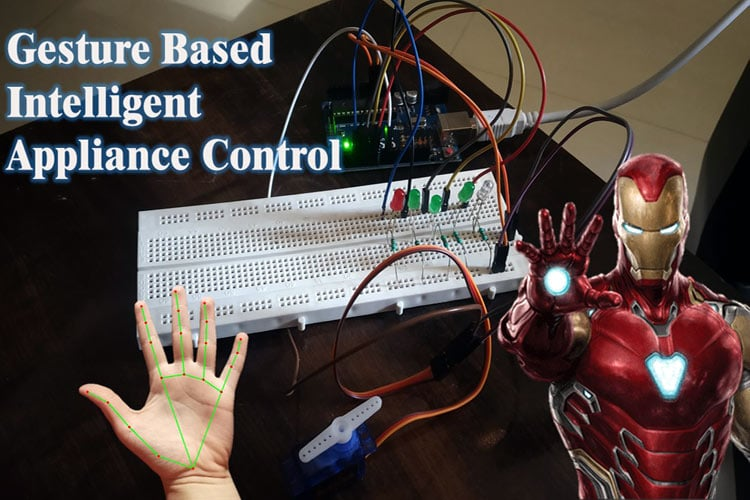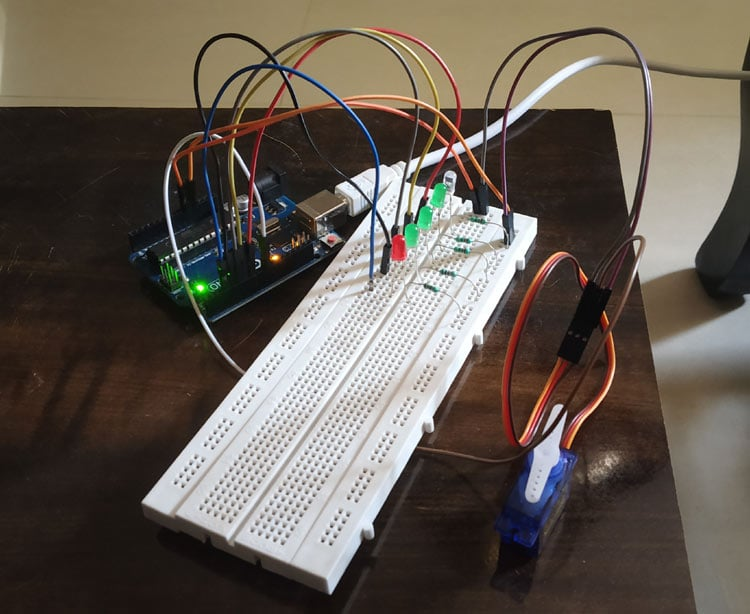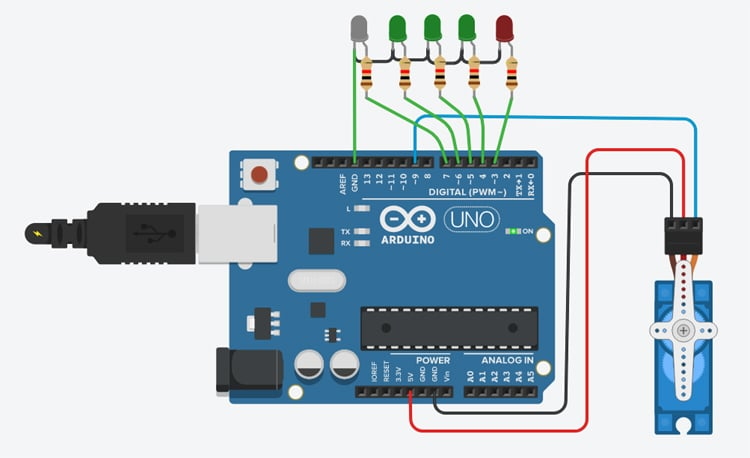1219 68
Manav Thakkar

## Gesture based Intelligent Appliance Control

With Artificial Intelligence, the science fiction is turning into reality and automation is taking...

##### Description:-

With Artificial Intelligence, the science fiction is turning into reality and automation is taking over the world. Inspired by the Iron man and Jarvis we decided to build something similar which can be controlled just by hand gestures. This system detects the hand and identifies the gesture with the help of artificial intelligence and based on that, it controls the appliances. It can be programmed to recognize different gestures and control the appliances accordingly. It can be used in a smart home to control AC appliances with just hand gestures, control the speed of the fan, control volume, control brightness of the lights, or even open/close the door stopper through gestures (with the use of servo motor), this can be very helpful for old age people who have limited mobility.### Project Used Hardware

Arduino Uno/Nano, LEDs, Micro-Servo Motor, Webcam (For prototype), 330-ohm resistors, Raspberry Pi (For prototype, Internal processor of PC is used)

### Project Used Software

Pycharm (OpenCV library, Mediapipe library, PySerial library), Python, Arduino IDE

### Project Hardware Software Selection

For prototype we have used LEDs and Servo Motor to demonstrate how it works, Arduino is selected because it is easily available and is open-source. It uses OpenCV and mediapipe library for real-time hand land mark detection from the webcam and based on that it returns the co-ordinates of the hand landmarks. Once the co-ordinates are obtained, some mathematical algorithms are applied to check how many fingers are raised (by that gesture is detected) and that number is stored in a variable. With the help of pyserial library it is passed to the Arduino serially, now with this information Arduino is programmed to perform certain tasks based on the information got from the python script. We used small LEDs for the demonstration, but AC appliances can also be controlled by the use of relay (electro-mechanical switches). For prototype version inbuilt micro-processor of laptop and webcam is used for real time tracking and gesture recognition, which can be replaced with a raspberry pi and a piCam at the final stage.

### Circuit Diagram

For this prototype, LEDs are connected to the pin number 3,4,5,6 and 7 respectively and a servo motor is connected to pin number 9. Relays can be connected in place of LEDs to control AC appliances. You can connect as many appliances as you want till you run out of pins or gestures.##### Code

``` ```

```# PYTHON CODE (Main code) import cv2 import time import HandTrackingModule as htm import serial # code reference from cvzone ser1 = serial.Serial('COM8',9600) #change according to your arduino COM port ser1.timeout = 1 wCam, hCam = 640, 480 cap = cv2.VideoCapture(0) cap.set(3, wCam) cap.set(4, hCam) pTime = 0 detector = htm.handDetector(detectionCon=0.75,maxHands=1) tipIds = [4, 8, 12, 16, 20] while True:     success, img = cap.read()     img = detector.findHands(img)     lmList = detector.findPosition(img, draw=False)     if len(lmList) != 0:         fingers = []         # Thumb         if lmList[tipIds] > lmList[tipIds - 1]:             fingers.append(1)         else:             fingers.append(0)         # 4 Fingers         for id in range(1, 5):             if lmList[tipIds[id]] < lmList[tipIds[id] - 2]:                 fingers.append(1)             else:                 fingers.append(0)         # print(fingers)         totalFingers = fingers.count(1)         print(100+totalFingers)         ser1.write(str(100+totalFingers).encode())         cv2.rectangle(img, (20, 225), (170, 425), (0, 255, 250), cv2.FILLED)         cv2.putText(img, str(totalFingers), (45, 375), cv2.FONT_HERSHEY_PLAIN,                     10, (255, 0, 0), 25)     cTime = time.time()     fps = 1 / (cTime - pTime)     pTime = cTime     cv2.putText(img, f'FPS: {int(fps)}', (400, 70), cv2.FONT_HERSHEY_PLAIN,                 3, (255, 0, 0), 3)```

```     cv2.imshow("Image", img)     cv2.waitKey(1) # ser1.close() //ARDUINO CODE include int incomingByte = 0; int num; Servo myservo; int pos = 0; void setup() {   // put your setup code here, to run once:   Serial.begin(9600);   myservo.attach(9);   pinMode(3,OUTPUT);   pinMode(4,OUTPUT);   pinMode(5,OUTPUT);   pinMode(6,OUTPUT);   pinMode(7,OUTPUT); } void loop() {   // put your main code here, to run repeatedly:  char sdata;  if (Serial.available()>0){   incomingByte = Serial.readBytes(sdata,3);    int d1 = sdata-'0';   int d2 = sdata-'0';   int d3 = sdata-'0'; //  if (d3==-38){ // because when 2 dig no. is entered d3 == -38 //    num = d1*10  +  d2; //    Serial.println(num); //    pos = map(num,100,105,0,170); //    myservo.write(pos); // //    if(num==101){ //      digitalWrite(3,1); //    } //    else{ //      digitalWrite(3,0); //    } // //  }   if(d3!=-38){   int num = d1*100  +  d2*10  +  d3;   if (num>0){   Serial.println(num);   pos = map(num,100,105,0,170);   myservo.write(pos);     if(num==101){        // switch case can be used       digitalWrite(3,1);       digitalWrite(4,0);       digitalWrite(5,0);       digitalWrite(6,0);       digitalWrite(7,0);     }     else if(num==102){       digitalWrite(3,1);       digitalWrite(4,1);       digitalWrite(5,0);       digitalWrite(6,0);       digitalWrite(7,0);           }     else if(num==103){       digitalWrite(3,1);       digitalWrite(4,1);       digitalWrite(5,1);       digitalWrite(6,0);       digitalWrite(7,0);           }     else if(num==104){       digitalWrite(3,1);       digitalWrite(4,1);       digitalWrite(5,1);       digitalWrite(6,1);       digitalWrite(7,0);           }     else if(num==105){       digitalWrite(3,1);       digitalWrite(4,1);       digitalWrite(5,1);       digitalWrite(6,1);       digitalWrite(7,1);           }        else{       digitalWrite(3,0);       digitalWrite(4,0);       digitalWrite(5,0);       digitalWrite(6,0);       digitalWrite(7,0);       }   }   }  } } #HAND TRACKING MODULE (put this in the same folder as the main code with name HandTrackingModule.py) #code from cvzone import cv2 import mediapipe as mp import time class handDetector():     def __init__(self, mode=False, maxHands=2, detectionCon=0.5, trackCon=0.5):         self.mode = mode         self.maxHands = maxHands         self.detectionCon = detectionCon         self.trackCon = trackCon         self.mpHands = mp.solutions.hands         self.hands = self.mpHands.Hands(self.mode, self.maxHands,                                         self.detectionCon, self.trackCon)         self.mpDraw = mp.solutions.drawing_utils     def findHands(self, img, draw=True):         imgRGB = cv2.cvtColor(img, cv2.COLOR_BGR2RGB)         self.results = self.hands.process(imgRGB)         # print(results.multi_hand_landmarks)         if self.results.multi_hand_landmarks:             for handLms in self.results.multi_hand_landmarks:                 if draw:                     self.mpDraw.draw_landmarks(img, handLms,                                                self.mpHands.HAND_CONNECTIONS)         return img     def findPosition(self, img, handNo=0, draw=True):         lmList = []         if self.results.multi_hand_landmarks:             myHand = self.results.multi_hand_landmarks[handNo]             for id, lm in enumerate(myHand.landmark):                 # print(id, lm)                 h, w, c = img.shape                 cx, cy = int(lm.x * w), int(lm.y * h)                 # print(id, cx, cy)                 lmList.append([id, cx, cy])                 if draw:                     cv2.circle(img, (cx, cy), 5, (255, 0, 255), cv2.FILLED)         return lmList def main():     pTime = 0     cTime = 0     cap = cv2.VideoCapture(0)     detector = handDetector()     while True:         success, img = cap.read()         img = detector.findHands(img)         lmList = detector.findPosition(img)         if len(lmList) != 0:             print(lmList)         cTime = time.time()         fps = 1 / (cTime - pTime)         pTime = cTime         cv2.putText(img, str(int(fps)), (10, 70), cv2.FONT_HERSHEY_PLAIN, 3,                     (255, 0, 255), 3)         cv2.imshow("Image", img)         cv2.waitKey(1) if __name__ == "__main__":     main() ```